# Speed Distance Time Speed Distance Time Calculations The

• Slides: 20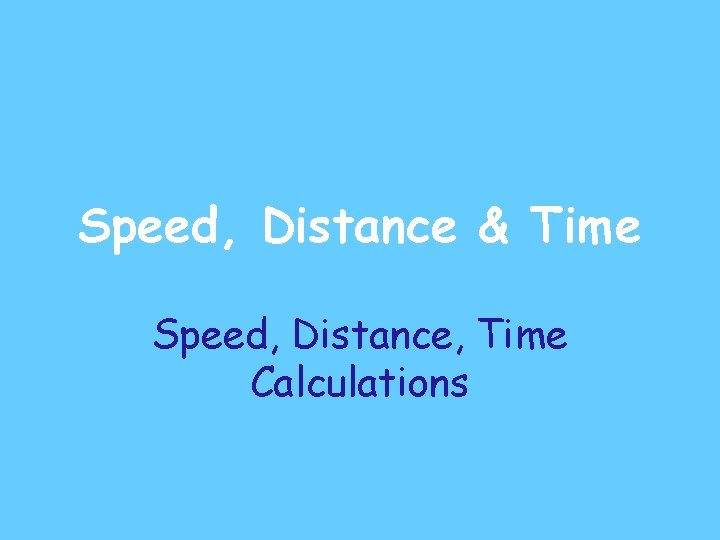Speed, Distance & Time Speed, Distance, Time Calculations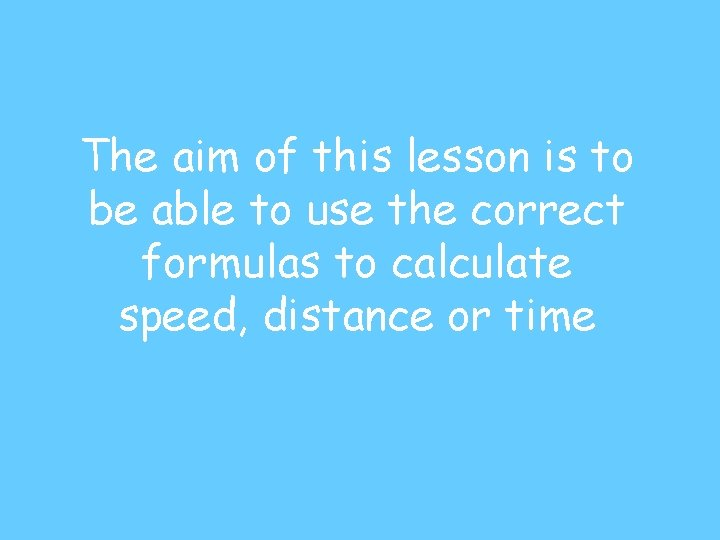The aim of this lesson is to be able to use the correct formulas to calculate speed, distance or timeConversion of Units 1000 mm 1 km = ? ? ? 60 min 1 hr = ? ? ? 100 cm cm 1 m = ? ? ? 60 sec 1 min = ? ? ? sec 10 mm 1 cm = ? ? ? mm 3600 sec 1 hr = ? ? ?Test yourself!!! m 2. 5 km = 2500 ? ? ? m 90 min 1. 5 hr = ? ? ? min 300 cm cm 3 m = ? ? ? 120 sec 2 min = ? ? ? 50 mm 5 cm = ? ? ? mm 3600 sec 1 hr = ? ? ?Reminder: How to change minutes into hour 15 mins = 0 • 25 hour (15 ÷ 60) So: 3 hours 15 mins = 3 • 25 hours 30 mins = 0 • 5 hour (30 ÷ 60) So: 7 hours 30 mins = 7 • 5 hours 45 mins = 0 • 75 hour (45 ÷ 60) So: 2 hours 45 mins = 2 • 75 hours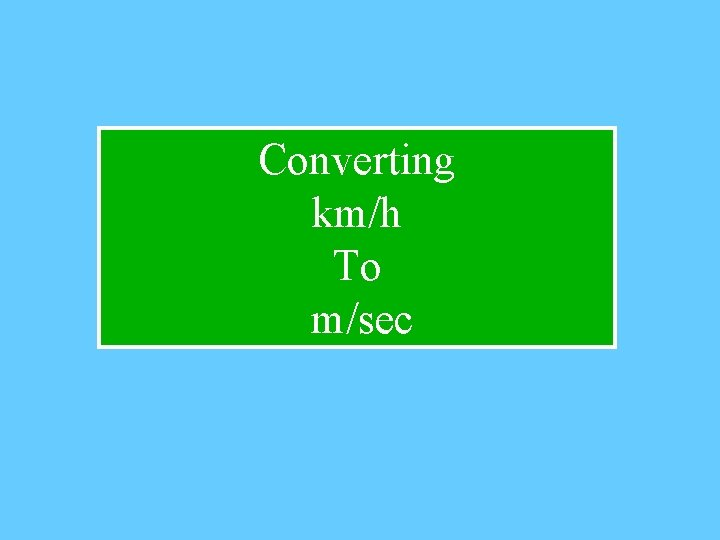Converting km/h To m/secEg 1: Convert 86 kilometres per hour (km/hr) into metres per second (m/sec) 1 km = 1000 m 86 km = 86 000 m m/sec = 86 000 m 3600 sec 1 hr = 3600 sec = 23 8 9 m/sec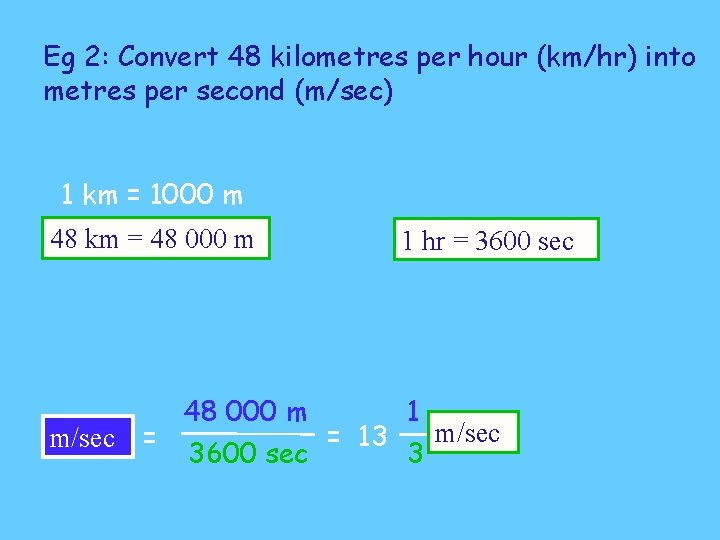Eg 2: Convert 48 kilometres per hour (km/hr) into metres per second (m/sec) 1 km = 1000 m 48 km = 48 000 m m/sec = 48 000 m 3600 sec 1 hr = 3600 sec = 13 1 3 m/secConverting m/sec To km/hr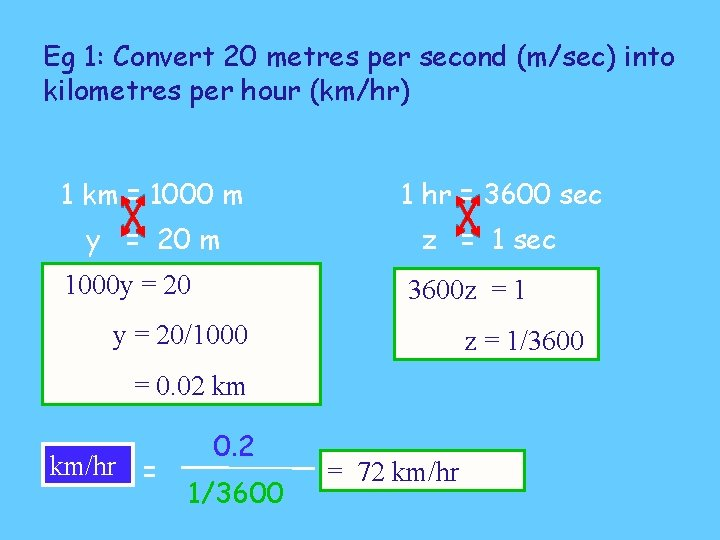Eg 1: Convert 20 metres per second (m/sec) into kilometres per hour (km/hr) 1 km = 1000 m y = 20 m 1000 y = 20 1 hr = 3600 sec z = 1 sec 3600 z = 1 y = 20/1000 z = 1/3600 = 0. 02 km km/hr = 0. 2 1/3600 = 72 km/hr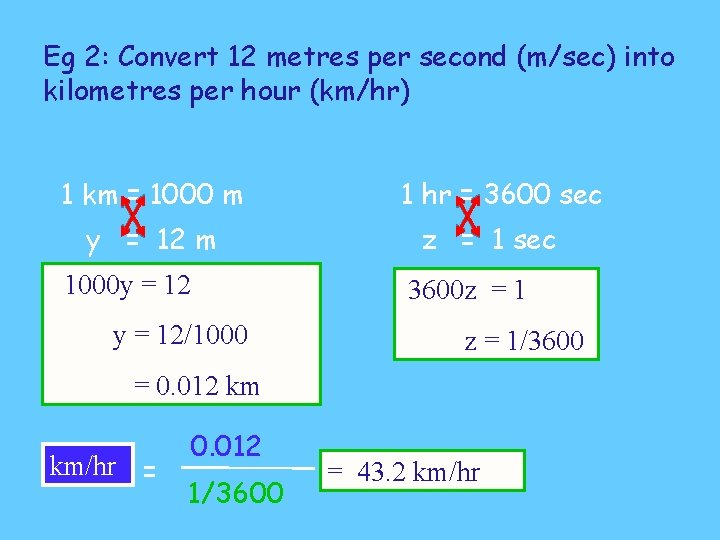Eg 2: Convert 12 metres per second (m/sec) into kilometres per hour (km/hr) 1 km = 1000 m y = 12 m 1000 y = 12/1000 1 hr = 3600 sec z = 1 sec 3600 z = 1/3600 = 0. 012 km km/hr = 0. 012 1/3600 = 43. 2 km/hr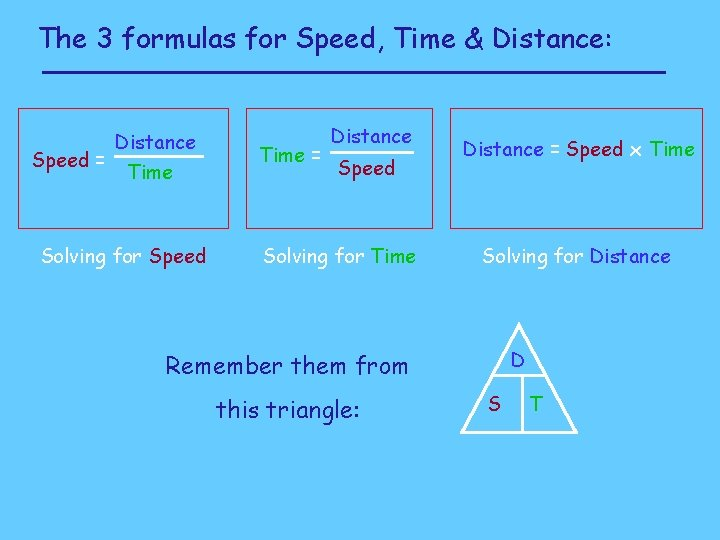The 3 formulas for Speed, Time & Distance: Distance Speed = Time Solving for Speed Time = Distance Speed Solving for Time Distance = Speed x Time Solving for Distance D Remember them from this triangle: S TThe 3 formulas for Speed, Time & Distance: Average Speed = Total Distance = Total Time = Total Distance Total Time Average Speed x Time Total Distance Average Speed D S T1) A windsurfer travelled 28 km in 1 hour 45 mins. Calculate his speed. Speed = = Distance Time 28 km 1 • 75 h D S T 1 hour 45 mins = 16 km/h Answer: His speed was 16 km / hour2) A salesman travelled at an average speed of 50 km/h for 2 hours 30 mins. How far did he travel? Distance = Speed x Time = 50 km/h x 2 • 5 hr D S T 2 hour 30 mins = 125 km Answer: He travelled 125 km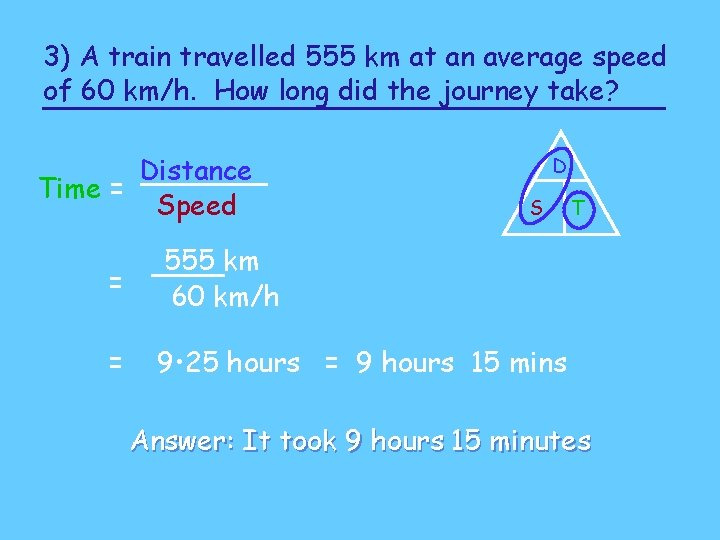3) A train travelled 555 km at an average speed of 60 km/h. How long did the journey take? Distance Time = Speed D S = 555 km 60 km/h = 9 • 25 hours = 9 hours 15 mins T Answer: It took 9 hours 15 minutes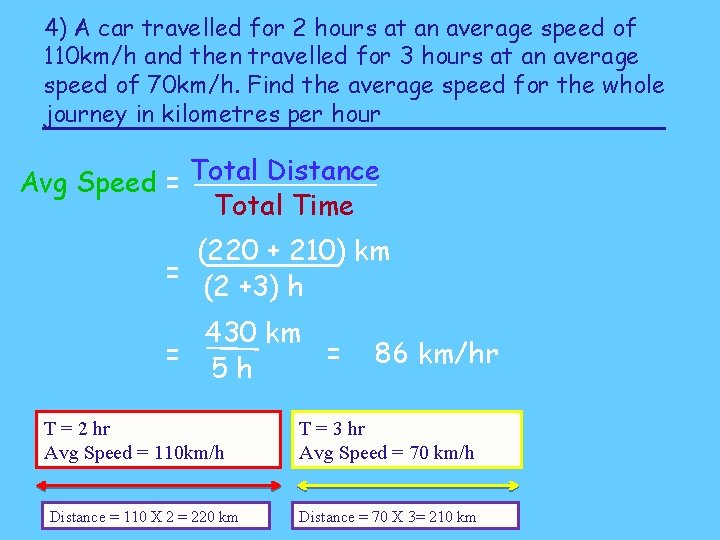4) A car travelled for 2 hours at an average speed of 110 km/h and then travelled for 3 hours at an average speed of 70 km/h. Find the average speed for the whole journey in kilometres per hour Avg Speed = Total Distance Total Time (220 + 210) km = (2 +3) h 430 km = = 5 h T = 2 hr Avg Speed = 110 km/h Distance = 110 X 2 = 220 km 86 km/hr T = 3 hr Avg Speed = 70 km/h Distance = 70 X 3= 210 km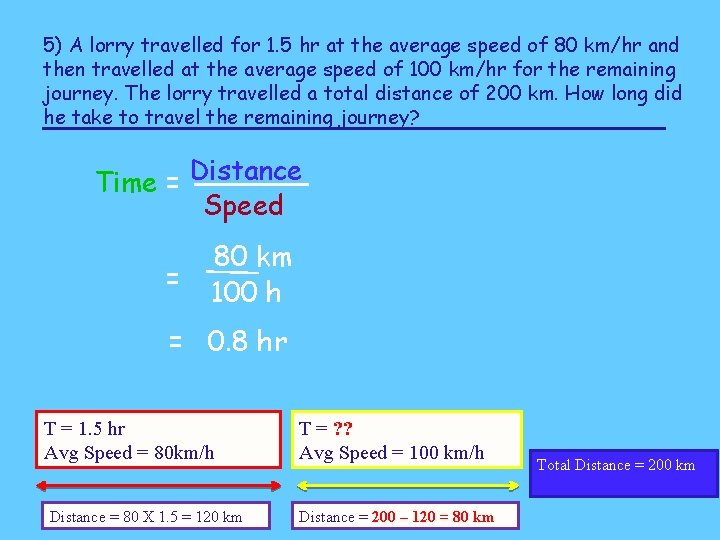5) A lorry travelled for 1. 5 hr at the average speed of 80 km/hr and then travelled at the average speed of 100 km/hr for the remaining journey. The lorry travelled a total distance of 200 km. How long did he take to travel the remaining journey? Time = Distance Speed = 80 km 100 h = 0. 8 hr T = 1. 5 hr Avg Speed = 80 km/h Distance = 80 X 1. 5 = 120 km T = ? ? Avg Speed = 100 km/h Distance = 200 – 120 = 80 km Total Distance = 200 kmConvert 86 kilometres per hour (km/hr) into metres per second (m/sec) 1 km = 1000 m 1 hr = 60 min 86 km = 86 000 m 1 min = 60 sec 1 hr = 3600 sec m/sec = 86 000 m 3600 sec = 23 8 9 m/sec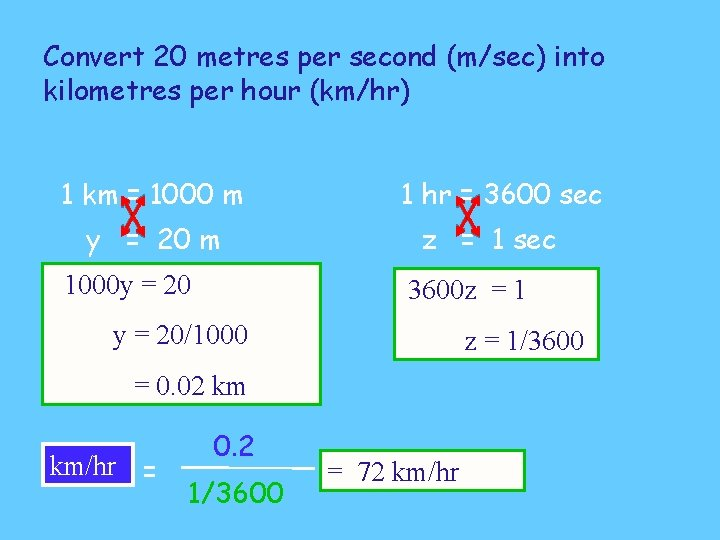Convert 20 metres per second (m/sec) into kilometres per hour (km/hr) 1 km = 1000 m y = 20 m 1000 y = 20 1 hr = 3600 sec z = 1 sec 3600 z = 1 y = 20/1000 z = 1/3600 = 0. 02 km km/hr = 0. 2 1/3600 = 72 km/hr